# The PDLREG Procedure

### Introductory Example

The following statements generate simulated data for variables Y and X. Y depends on the first three lags of X, with coefficients .25, .5, and .25. Thus, the effect of changes of X on Y takes effect 25% after one period, 75% after two periods, and 100% after three periods.

data test;
xl1 = 0; xl2 = 0; xl3 = 0;
do t = -3 to 100;
x = ranuni(1234);
y = 10 + .25 * xl1 + .5 * xl2 + .25 * xl3
+ .1 * rannor(1234);
if t > 0 then output;
xl3 = xl2; xl2 = xl1; xl1 = x;
end;
run;


The following statements use the PDLREG procedure to regress Y on a distributed lag of X. The length of the lag distribution is 4, and the degree of the distribution polynomial is specified as 3.

proc pdlreg data=test;
model y = x( 4, 3 );
run;


The PDLREG procedure first prints a table of statistics for the residuals of the model, as shown in Figure 21.1. See Chapter 8: The AUTOREG Procedure, for an explanation of these statistics.

Figure 21.1: Residual Statistics

The PDLREG Procedure

Dependent Variable y

Ordinary Least Squares Estimates
SSE 0.86604442 DFE 91
MSE 0.00952 Root MSE 0.09755
SBC -156.72612 AIC -169.54786
MAE 0.07761107 AICC -168.88119
MAPE 0.73971576 HQC -164.3651
Durbin-Watson 1.9920 Regress R-Square 0.7711
Total R-Square 0.7711

The PDLREG procedure next prints a table of parameter estimates, standard errors, and t tests, as shown in Figure 21.2.

Figure 21.2: Parameter Estimates

Parameter Estimates
Variable DF Estimate Standard
Error
t Value Approx
Pr > |t|
Intercept 1 10.0030 0.0431 231.87 <.0001
x**0 1 0.4406 0.0378 11.66 <.0001
x**1 1 0.0113 0.0336 0.34 0.7377
x**2 1 -0.4108 0.0322 -12.75 <.0001
x**3 1 0.0331 0.0392 0.84 0.4007

The table in Figure 21.2 shows the model intercept and the estimated parameters of the lag distribution polynomial. The parameter labeled X**0 is the constant term,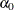, of the distribution polynomial. X**1 is the linear coefficient,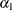; X**2 is the quadratic coefficient,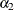; and X**3 is the cubic coefficient,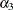.

The parameter estimates for the distribution polynomial are not of interest in themselves. Since the PDLREG procedure does not print the orthogonal polynomial basis that it constructs to represent the distribution polynomial, these coefficient values cannot be interpreted.

However, because these estimates are for an orthogonal basis, you can use these results to test the degree of the polynomial. For example, this table shows that the X**3 estimate is not significant; the p-value for its t ratio is 0.4007, while the X**2 estimate is highly significant (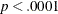). This indicates that a second-degree polynomial might be more appropriate for this data set.

The PDLREG procedure next prints the lag distribution coefficients and a graphical display of these coefficients, as shown in Figure 21.3.

Figure 21.3: Coefficients and Graph of Estimated Lag Distribution

Estimate of Lag Distribution
Variable Estimate Standard
Error
t Value Approx
Pr > |t|

-0.04                                0.4167
x(0) -0.040150 0.0360 -1.12 0.2677 |***|                                     |
x(1) 0.324241 0.0307 10.55 <.0001 |   |*****************************        |
x(2) 0.416661 0.0239 17.45 <.0001 |   |*************************************|
x(3) 0.289482 0.0315 9.20 <.0001 |   |**************************           |
x(4) -0.004926 0.0365 -0.13 0.8929 |   |                                     |

The lag distribution coefficients are the coefficients of the lagged values of X in the regression model. These coefficients lie on the polynomial curve defined by the parameters shown in Figure 21.2. Note that the estimated values for X(1), X(2), and X(3) are highly significant, while X(0) and X(4) are not significantly different from 0. These estimates are reasonably close to the true values used to generate the simulated data.

The graphical display of the lag distribution coefficients plots the estimated lag distribution polynomial reported in Figure 21.2. The roughly quadratic shape of this plot is another indication that a third-degree distribution curve is not needed for this data set.